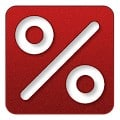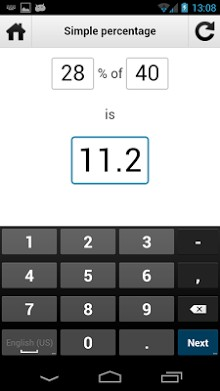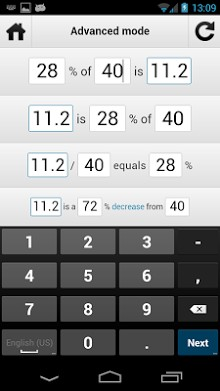# Percentage Calculator v1 APKPercentage Calculator is simply the best and easiest to use app which calculates:
* simple percentage calculator (5 percent of 40 is 2)
* percentage increase/decrease (5 percent decrease from 40 is 38)
* restaurant tip amount
* discount rate
* markup
* profit margin
* convert fractions to percentages (5 / 20 is the same as 25 percent)
Percentage calculator is unique in a way that it calculates everything on the fly (no need to press any “submit button”) and it lets you find out any part of the equasion, for example in “5% of 40 is 2” you could have entered any two of these three numbers and third one would be calculated for you. And that is what makes this percentage calculator stand out in the crowd!
Percentage calculator is useful in many real-life situations:
* school (mathematics, statistics, algebra)
* finances and business (investment return rate, loan interest rate, stock exchange quotes, markup, profit margin, company profits changes)
* shopping (discounts)
* tipping
* cooking (ingredients are often shown in percentages)
* health (body mass index, fat percentage in food)
This app is an expansion of the original percentage calculator web app which can be found on http://percentagecalculator.info .